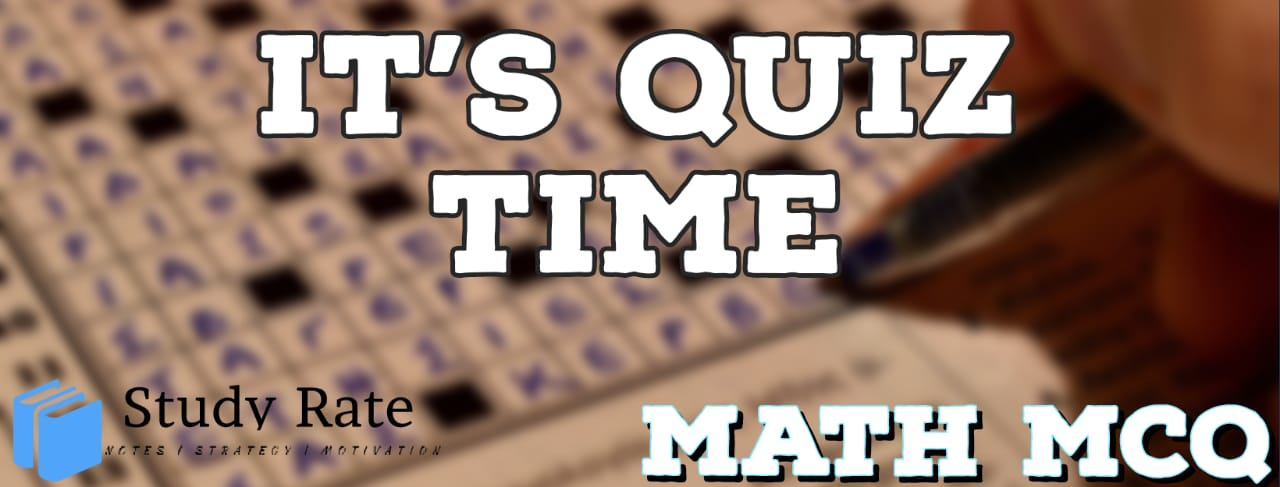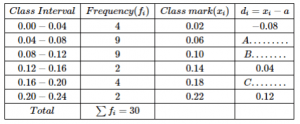## CBSE Class 10 Maths Chapter 14 – Statistics MCQ Quiz Online

Free PDF Download of CBSE Class 10 Maths Chapter 14 – Statistics Multiple Choice Questions with Answers. MCQ Questions for Class 10 Maths with Answers was Prepared Based on Latest Exam Pattern. Students can solve Class 10 Maths Statistics MCQs with Answers to know their preparation level

CBSE Board Class 10 Maths Chapter 14- Statistics Objective Questions

1. Class mark of a class is obtained by using

2. Look at the following table belowThe value of A & B respectively are:

3. Consider the following distribution of SO2 concentration in the air (in ppm = parts per million) in 30 localities. Find the mean SO2 concentration using assumed mean method. Also find the values of A, B and C.4. The average weight of a group of 25 men was calculated to be 78.4 kg. It was discovered later that one weight was wrongly entered as 69 kg instead of 96 kg. What is the correct average?

5. (For an arranged data) The median is the

6. For one term, absentee record of students is given below. If mean is 15.5, then the missing frequencies x and y are:7. For the following distribution,The sum of lower limits of median class and modal class is:

8. If the arithmetic mean of x, x+3, x+6, x+9 and x+12 is 10, then x = ?

9. If the mean of first n natural numbers is 5n/9, then n =?

10. The Median when it is given that mode and mean are 8 and 9 respectively, is: